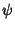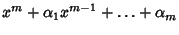## Gauss's Polynomial Theorem

If a Polynomialwith integral Coefficients is divisible into a product of two Polynomialsthen the Coefficients of this Polynomial are Integers.

See also Abel's Irreducibility Theorem, Abel's Lemma, Kronecker's Polynomial Theorem, Polynomial, Schoenemann's Theorem

References

Dörrie, H. 100 Great Problems of Elementary Mathematics: Their History and Solutions. New York: Dover, p. 119, 1965.